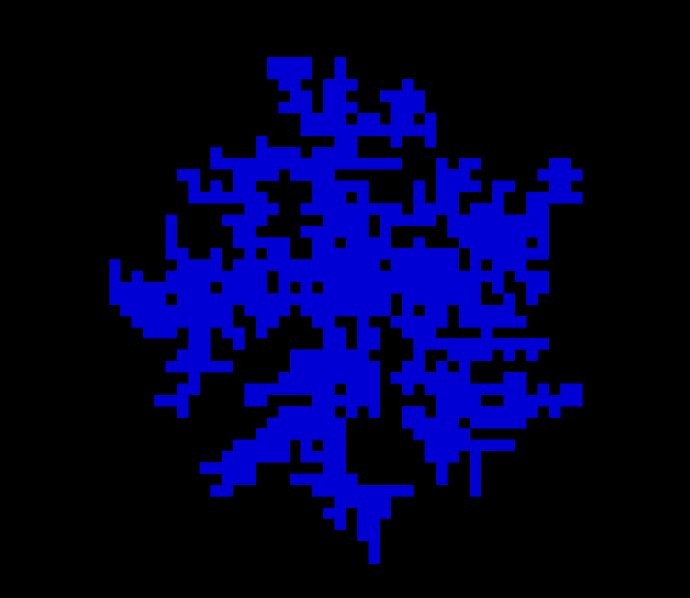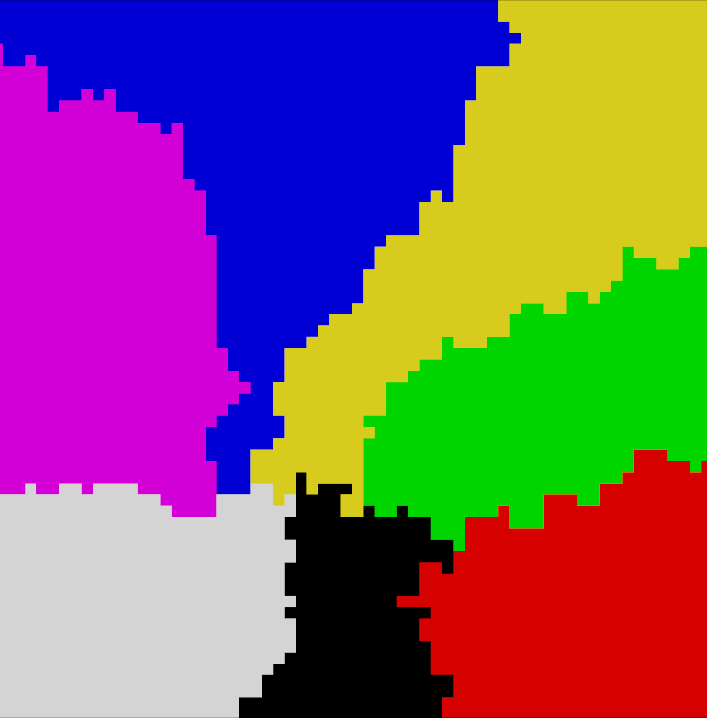# How to use the Diffusion Aggregation algorithm to generate 2D maps for dungeons or biomes

Published

Continuing on our series of articles exploring algorithms for procedural generation for Game Development, we’ll take a look at the diffusion aggregation algorithm. This algorithm can serve as both a generator for dungeon maps, similar to the Drunkard Walk (or Random Walk) but also it can serve to perform a partition or subdivision of a grid, making it an interesting alternative to Voronoi Diagrams.

## What is the Diffusion Aggregation algorithm

The Diffusion Aggregation algorithm, also known as Diffusion-Limited Aggregation (or DLA) algorithm is a computational model used to simulate the growth of “aggregates”. In plain terms, this means that the Diffusion Aggregation process starts from an initial area or shape in a grid and grows by adding new points connected to the initial area. This initial area is also called a “seed” and impacts the generation process.

## Why use the Diffusion Aggregation algorithm

The Diffusion Aggregation algorithm is simple to implement since it consists in simply moving particles on a grid and tracking collisions. For this reason it is also quite fast in its basic form, as it only requires tracking a single particle at a time.

The main reason to use the Diffusion Aggregation algorithm is for its results: it creates branching, fractal patterns which make for very interesting maps. Just like the Random Walk algorithm, it is suited for generating seemingly naturally created structures, such as grottos. These structures are also guaranteed to be fully connected.

## How does the Diffusion Aggregation algorithm work

The Diffusion Aggregation Algorithm follows a few simple steps:

1. Create a grid filled with 0s
2. Create the initial seed and set the relevant cells as 1 in the grid
3. Create a particle
4. Move this particle to a random neighbouring cell
5. Repeat 4. until the particle reaches a non-empty cell in the grid (the particle collides with the existing shape being created)
6. Set the position prior to the collision as 1 in the grid
7. Go back to 3. and repeat until the termination condition has been met.

It’s good to note that we use a random (or stochastic) movement in a 2D setup for our particles, but you can also use more complex non-stochastic models and even extend it to 3D.

## How to generate a 2D map with the diffusion aggregation algorithm in C# for Unity

As explained in the previous section, the algorithm is a grid based process so we’ll need to provide a size argument for our map, as well as implement a termination condition.

In our implementation, we’ll be using the number of steps as our termination condition. The following code closely follows the instructions we provided: it creates a 0-filled grid with a target size, finds the center of the grid and creates the initial seed (a square of length 3 here) then starts producing and moving particles. The direction for the particle’s movement is chosen at random at every iteration for its movement, so this is a random / stochastic process.

Here’s our code, annotated with comments:

``````public int[,] GenerateMap(Vector2Int size_map, int nb_steps)
{
int[,] grid = new int[size_map.x, size_map.y];

// create a tracker for allocated points for the diffusion process
List<Vector2Int> recorded_points = new();

// Diffusion process moves from a given point to another point in a one cell movement so we're setting the directions here as a reusable element
Vector2Int[] directions = new Vector2Int { Vector2Int.left, Vector2Int.up, Vector2Int.right, Vector2Int.down };

// set a initial seed, a cluster of tiles at the center of the room
Vector2Int center_map = new(
size_map.x / 2,
size_map.y / 2
);

for (int i = -1; i < 2; i++) {
for (int j = -1; j < 2; j++) {
Vector2Int temp = center_map + new Vector2Int(i, j);
grid[
temp.x,
temp.y
] = 1;
}
}

// The diffusion process consists in firing from a random point in a random direction until we hit a previously allocated point
System.Random rng = new();
for (int i = 0; i < nb_steps; i++)
{
// choose a random point
Vector2Int origin_point = new(
rng.Next(size_map.x),
rng.Next(size_map.y)
);

// move in a random direction until we hit something, or get out of bounds
while (true)
{
// choose a direction
Vector2Int direction = directions[rng.Next(4)];

Vector2Int new_point = origin_point + direction;

// check if the new point is in the grid. If it isn't, go to next step
if (new_point.x < 0 || new_point.y < 0 || new_point.x >= size_map.x || new_point.y >= size_map.y)
{
break;
}

// check if this is not a previously visited point
// if it is a visited point, we can add the "origin_point" to our grid
if (grid[new_point.x, new_point.y] == 1)
{
grid[origin_point.x, origin_point.y] = 1;
break;
}

// else we update origin point and continue moving our particle
origin_point = new_point;
}
}

return grid;
}``````

And we’re done! The Diffusion Aggregation algorithm is quite simple to implement but the results are quite interesting, let’s take a look:Sample generations of a 2D map using the diffusion aggregation algorithm

In our implementation we used the number of steps as the termination condition and I think the results are pretty neat. Still, there are a couple of other termination conditions you could use:

• Aggregate size, don’t stop the algorithm until it reaches a given size
• Connectivity level, the maps generated by this algorithm are usually branching which may mean a dead-ends and backtracking for the player. You can keep running the algorithm until cells have at least N connections on average, or inject corridors or other shapes in the grid.

## How to subdivide or partition a 2D grid with the diffusion aggregation algorithm

It’s also possible to modify the diffusion aggregation algorithm to use it as a partitioning / subdividing algorithm, which can then be used to define game elements such as biomes.

In this case, we want to divide a grid into multiple subsections, each with its own ID. We can accomplish this in a few steps:

1. Create a grid
2. Create initial points for each subsection, write them to the grid and store them in a tracker
3. For each subsection, choose a stored point
4. Choose a random direction
5. Get the neighbouring cell of the stored point using this direction
6. If this point in the grid is unallocated (i.e. has a value of -1), set the id of the subsection in the grid at the point’s location and add the point to the tracker
7. Continue from 3 with the next subsection
8. Repeat until the full grid has been filled

This algorithm will split a grid between different subsections and ensure that subsections are fully connected (no isolated child for any subsection).

``````public int[,] GeneratePartitionedMap(Vector2Int size_map, int nb_subsections)
{
int[,] grid = new int[size_map.x, size_map.y];

// fill with -1s to get the initial state
for (int i = 0; i < size_map.x; i++)
{
for (int j = 0; j < size_map.y; j++)
{
grid[i, j] = -1;
}
}

// create a tracker for valid points by subsection for the diffusion process
List<List<Vector2Int>> recorded_points = new();
for (int i = 0; i < nb_subsections; i++)
{
}

// create a counter to keep track of the number of unallocated cells
int nb_free_cells = size_map.x * size_map.y;

// set up the initial points for the process
System.Random rng = new();
for (int id_subsection = 0; id_subsection < nb_subsections; id_subsection++)
{
while (true)
{
// find a random point
Vector2Int point = new(
rng.Next(size_map.x),
rng.Next(size_map.y)
);

// check if it's free, else find another point
if (grid[point.x, point.y] == -1)
{
// if it is add it to tracking and grid then proceed to next subsection
grid[point.x, point.y] = id_subsection;
nb_free_cells -= 1;

break;
}
}
}

// Diffusion process moves from a given point to another point in a one cell movement so we're setting the directions here as a reusable element
Vector2Int[] directions = new Vector2Int { Vector2Int.left, Vector2Int.up, Vector2Int.right, Vector2Int.down };

// now we can start filling the grid
while (nb_free_cells > 0)
{
for (int id_subsection = 0; id_subsection < nb_subsections; id_subsection++)
{
// check if there are tracked points for this subsection
if (recorded_points[id_subsection].Count == 0)
{
continue;
}

// choose a random point from the tracked points
int id_curr_point = rng.Next(recorded_points[id_subsection].Count);

Vector2Int curr_point = recorded_points[id_subsection][id_curr_point];

// choose a direction at random
Vector2Int new_point = curr_point + directions[rng.Next(4)];

// check if the new point is in the grid
if (new_point.x < 0 || new_point.y < 0 || new_point.x >= size_map.x || new_point.y >= size_map.y)
{
continue;
}

// next check if the new point is already occupied and skip this direction if it is
if (grid[new_point.x, new_point.y] != -1)
{
continue;
}

// else we can record this new point in our tracker and set it in the grid
grid[new_point.x, new_point.y] = id_subsection;
nb_free_cells -= 1;

}
}

return grid;
}
``````

And here’s the result:Sample generations of a 2D map using a modified diffusion aggregation algorithm for grid partitioning

As I mentioned previously, a prime application of this type of algorithm is to create biomes. And actually we can compare the result to another algorithm that is commonly used for this kind of purposes: Voronoi Diagrams.